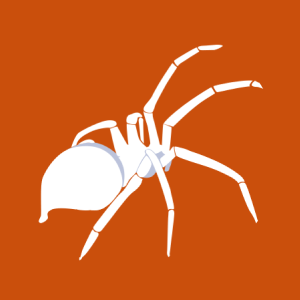# PHP-Nuke Modules Okul 1.0 okulid Remote SQL Injection Vulnerability

2008-02-20T00:00:00
ID EDB-ID:5159
Type exploitdb
Reporter Mehmet Ince
Modified 2008-02-20T00:00:00

## PHP-Nuke Modules Okul 1.0 (okulid) Remote SQL Injection Vulnerability. CVE-2008-0881. Webapps exploit for php platform

```                                        ```
=-==-==-==-==-==-==-==X==O==R==O==N==-==-==-==-==-==-==-==-==-==-==-=

PHP-NUKE Modules Okul v1.0 Remote SQL Injection

=-==-==-==-==-==-==-==X==O==R==O==N==-==-==-==-==-==-==-==-==-==-==-=

Found: xoron

contact: xorontr@gmail.com (only e-mail)

=-==-==-==-==-==-==-==X==O==R==O==N==-==-==-==-==-==-==-==-==-==-==-=

Exploit: